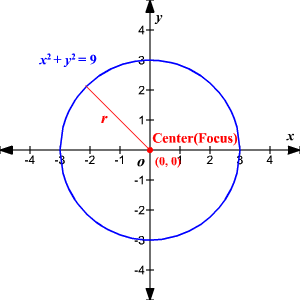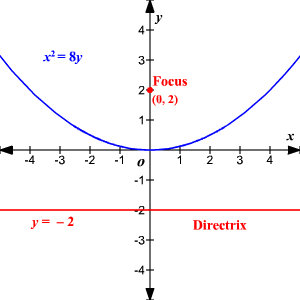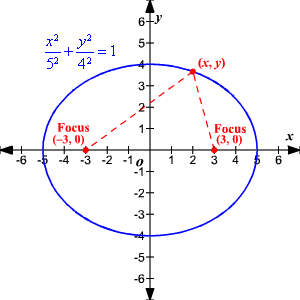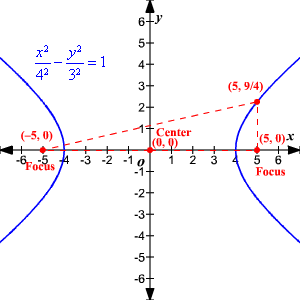# Focus

A focus is a point used to construct a conic section.  (The plural is foci .)  The focus points are used differently to determine each conic.

A circle is determined by one focus. A circle is the set of all points in a plane at a given distance from the focus (center).A parabola is determined by a focus and a directrix (a line).  A parabola is the set of points in a plane such that the distance from the focus equals the distance to the directrix.An ellipse is determined by two foci.  An ellipse is the set of points in a plane such that the sum of the distance from a point to each focus is constant.A hyperbola is determined by two foci.  A hyperbola is the set of points in a plane such that the difference between the distances from a point to each focus is constant.